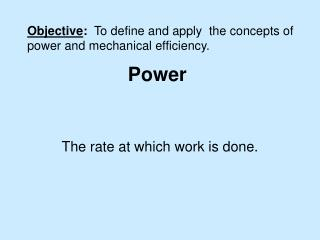DownloadDownload PresentationPower

# Power

Télécharger la présentation## Power

- - - - - - - - - - - - - - - - - - - - - - - - - - - E N D - - - - - - - - - - - - - - - - - - - - - - - - - - -
##### Presentation Transcript

1. Objective: To define and apply the concepts of power and mechanical efficiency. Power The rate at which work is done.

2. The power is the work done divided by the time it takes to do the work. Units: J/s = Watt 1 horsepower =746 Watts 1000 Watts = 1 kW Kilowatt-hours (kwh) measure energy.

3. SRP charges about 10 cents for 1kwh. How much would it cost to leave a 60W light bulb on for a full day? Leaving a 60W light bulb on for 24 hours will cost about 15 cents.

4. This equation can be written in another form:

5. Which has more power?

6. Work is transferred to gravitational potential Energy A 60kg man takes 5 seconds to run up a set of stairs at a constant velocity. What was the man’s power? Mass = 60kg 15m

7. f F A man pushes a 12kg crate horizontally with a constant velocity of 2m/s. The coefficient of friction between the crate and the floor is 0.25. What power does the man have as he pushes the crate? 120N -120N

8. A crane hoist lifts a load of 1000kg a vertical distance of 25m in 9.0s at a constant velocity. What is the useful power output of the crane?

9. If the hoist motor of the previous crane is rated at 70hp, what percentage of this power goes to useful work?

10. Efficiency – A measure of what you get out for what you put in. The Efficiency of a typical car is about 25%!

11. The motor of an electric drill with an efficiency of 80% has a power of input of 600 W. How much useful work is done by the drill in a time of 30 seconds?